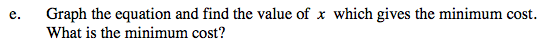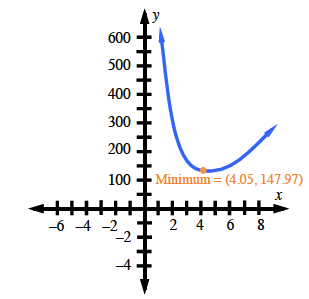### Home > CAAC > Chapter 16 > Lesson 16.9.1.3 > Problem9-39

9-39.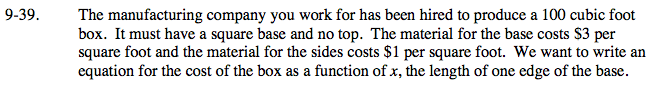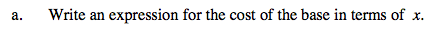Write and expression for the area and multiply that by the cost per square foot.

Area: x2
Cost: 3x2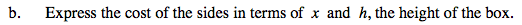Write an expression for the area of one side.
Write an expression for the area of four sides.
Write an expression for the cost of four sides.

Area: 4xh
Cost: (1)(4xh) = 4xh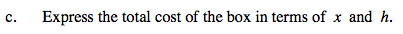This is the sum of your expressions from parts (a) and (b).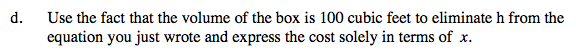100 = x2h

$h = \frac{100}{x^2}$

Substitute 'h' into the equation in part (c).